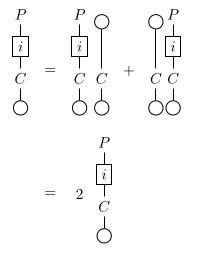# nLab primitive element

Contents

### Context

#### Algebra

higher algebra

universal algebra

# Contents

## Definition

Working over a commutative ring $R$, recall that a unital or coaugmented coalgebra is an $R$-coalgebra $(C, \Delta: C \to C \otimes_R C, \epsilon: C \to R)$ equipped with a coalgebra map $u: R \to C$. Abusing notation, denote $u(1) \in C$ by $1$.

### Primitive element in a coalgebra

An element $x$ in a coalgebra $C$ is primitive if $\Delta(x) = 1\otimes x + x\otimes 1$. This condition implies $\epsilon(x) = 0$.

This notion generalizes straightforwardly to unital corings over $R$ and even more generally to any unital comonoid object in a CMon-enriched monoidal category (see below).

### Primitive element in a comodule

Let $N$ be a comodule over $C$, with co-action map $\Psi \colon N \longrightarrow C \otimes_R N$. Then an element $n \in N$ is called a primitive element or coinvariant if $\Psi(n) = 1 \otimes n$.

The $R$-module $prim(N)$ of primitive elements of $N$ is naturally to

1. the module of comodule homomorphisms out of $R$ into $N$

$prim(N) \simeq Hom_{C}(R,N) \,.$
2. the cotensor product of $N$ with $A$

$prim(N) \simeq A \Box_R N \,.$

### Object of primitive elements of a unital comonoid in a $CMon$-enriched monoidal category

Let $(C,\Delta,\epsilon)$ be a unital comonoid object in a CMon-enriched monoidal category $(\mathcal{C},\otimes,I)$. The object $P$ of primitive elements of $C$ is defined if it exists, as the equalizer in this diagram:

###### Proposition

If the unital comonoid $C$ admits an object $P$ of primitive elements, the commutative rig $\mathcal{C}[I,I]$ verifies $2 \neq 1$, and the homsets $\mathcal{C}$[A,B] are multiplicatively cancellative modules over $\mathcal{C}[I,I]$, we then have:

###### Proof

In this diagram, the path from $C$ to $C$ by the upper square (with below side equal to $\Delta$) is equal to the identity and the two paths from $P$ to $C \otimes C$ are equal:

We thus have that:

$i = i;\Delta;1 \otimes \epsilon = i;(1 \otimes \eta + \eta \otimes 1);(1 \otimes \epsilon)$

and:

$i;\epsilon = i;\Delta;1 \otimes \epsilon;\epsilon = i;(1 \otimes \eta + \eta \otimes 1);(1 \otimes \epsilon);\epsilon$
$= i;(1 \otimes \eta);(1 \otimes \epsilon);\epsilon + i;(\eta \otimes 1);(1 \otimes \epsilon);\epsilon$

We then have:$\mathcal{C}[P,I]$ being a multiplicatively cancellative $\mathcal{C}[I,I]$-module, if $i;\epsilon \neq 0$, we would have that $1 = 2$ in $\mathcal{C}[I,I]$ which is false, therefore $i;\epsilon = 0$.

## Properties

### Primitives in a Hopf algebra

A straightforward calculation shows that the module of primitive elements in a Hopf algebra $H$ (or even in a bialgebra $H$) is a Lie subalgebra of the underlying Lie algebra of $H$ (whose bracket is the algebra commutator). Thus, taking primitive elements yields a functor

$P: HopfAlg \to LieAlg$

(and of course we have more generally a functor $P: BiAlg \to LieAlg$ which is an extension along the full inclusion $HopfAlg \to BiAlg$).

For a Lie algebra $L$, let $U(L)$ be its universal enveloping algebra:

$U(L) = T(L)/I$

where $I$ is the two-sided ideal generated by elements of the form $x y - y x - [x, y]$ where $x, y \in L$. This carries a bialgebra structure whose comultiplication $\delta: U(L) \to U(L) \otimes U(L)$ is uniquely determined by the rule $\delta(x) = 1 \otimes x + x \otimes 1$ for $x \in L$. Since this says $x \in L$ is primitive, the counit $\epsilon: U(L) \to R$ is forced to be the algebra map such $\epsilon(x) = 0$ for all $x \in L$, and also the Hopf antipode is uniquely determined: $\sigma(x) = -x$ for $x \in L$.

The following proposition is entirely straightfoward:

###### Proposition

The functor $U: LieAlg \to BiAlg$ is left adjoint to the functor $P: BiAlg \to LieAlg$.

This result is essentially tautologous and holds for any commutative ring of arbitrary characteristic. (This despite the fact that the $U(L)$ as defined above is not as well-behaved in nonzero characteristic as one might like; e.g. the PBW theorem fails.) More information on this adjunction may require more restrictive hypotheses:

###### Proposition

For $R = k$ a field of characteristic zero, the unit $L \to P U(L)$ is an isomorphism.

An immediate consequence is that for such ground fields $k$, the functor $U: LieAlg \to BiAlg$ is fully faithful. Of course, $U$ lands in the full subcategory of cocommutative Hopf algebras, which is exactly the category of group objects in the cartesian monoidal category of cocommutative coalgebras.

The Milnor-Moore theorem gives further information: for Hopf algebras over a field of characteristic zero, the counit $U P(H) \to H$ is a monomorphism, and an isomorphism in case $H$ satisfies a suitable conilpotency condition.